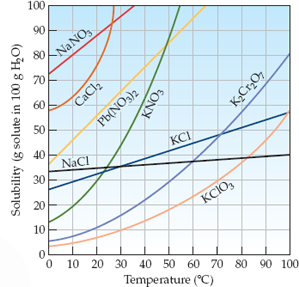×
Log in to StudySoup
Get Full Access to Introductory Chemistry - 5 Edition - Chapter 13 - Problem 35p
Join StudySoup for FREE
Get Full Access to Introductory Chemistry - 5 Edition - Chapter 13 - Problem 35p

Already have an account? Login here
×
Reset your password

# Refer to Figure 1 determine whether each of the given amounts of solid will completelyISBN: 9780321910295 34

## Solution for problem 35P Chapter 13

Introductory Chemistry | 5th Edition

• Textbook Solutions
• 2901 Step-by-step solutions solved by professors and subject experts
• Get 24/7 help from StudySoup virtual teaching assistantsIntroductory Chemistry | 5th Edition

4 5 1 355 Reviews
24
1
Problem 35P

Problem 35P

Refer to Figure 1 determine whether each of the given amounts of solid will completely dissolve in the given amount of water at the indicated temperature.

(a) 30.0 g KClO3 in 85.0 g of water at 35 °C

(b) 65.0 g NaNO3 in 125 g of water at 15 °C

(c) 32.0 g KCl in 70.0 g of water at 82 °C

Figure 1 Solubility of some ionic solids as a function of temperatureStep-by-Step Solution:
Step 1 of 3

Problem 35P

Refer to Figure 1 determine whether each of the given amounts of solid will completely dissolve in the given amount of water at the indicated temperature.

(a) 30.0 g KClO3 in 85.0 g of water at 35 °C

(b) 65.0 g NaNO3 in 125 g of water at 15 °C

(c) 32.0 g KCl in 70.0 g of water at 82 °C

Figure 1 Solubility of some ionic solids as a function of temperatureSolution: Here, we are going to identify if the given amounts of solid will dissolve completely in the given amount of water at the indicated temperature.

Step1:

Solubility of a substance is its maximum amount that can be dissolved in a specified amount of solvent. It depends upon the nature of solute and solvent as well as temperature and pressure.

Step2:

1. From figure 1, it is clear that the solubility of KClO3 at 35oC is about 11 g per 100 g of water.

Thus, 30.0 g of  KClO3 will not dissolve in 85.0 g water at 35oC.

b)        From figure 1, it is clear that the solubility of NaNO3 at 15oC is about 85 g per 100 g of

water.

Thus, 65.0 g of  NaNO3 will completely dissolve in 125 g water at 15oC.

c)        From figure 1, it is clear that the solubility of KCl at 82oC is about 51 g per 100 g of

water.

So, for dissolving 32.0 g of KCl, amount of water required = (100/51) x 32 = 63 g water

Thus, 32.0 g of  KCl will completely dissolve in 70.0 g water at 82oC.

------------------------------

Step 2 of 3

Step 3 of 3

##### ISBN: 9780321910295

Unlock Textbook Solution

Enter your email below to unlock your verified solution to:

Refer to Figure 1 determine whether each of the given amounts of solid will completely